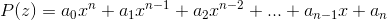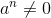# The Fundamental Theorem of Algebra

SprucerMoose
Hi all,
I'm currently flicking through and old textbook and came accross the following.

"Every polynomial of the form, wherehas n linear factors over C...". What does it mean by [URL]http://latex.codecogs.com/gif.latex?a^{n}\neq0?[/URL] Is this referring to some kind of complex index? This is all that is written and nothing precedes it. I just dont quite understand the.

Edit: Latex kind of fixed

Last edited by a moderator:

Homework Helper
Hi SprucerMoose!(try using the X2 and X2 icons just above the Reply box)

it must be a misprint for "where a0 ≠ 0" …

in other words, where it's a genuine nth-degree polynomialSprucerMoose
Ah, I see. Thank you very much.

Testing...

a2 + a0 + a1

Edit: It works!

Homework Helper
Testing...

a2 + a0 + a1

Edit: It works!

Now you really are sprucer!Mentor
Ah, I see. Thank you very much.

Testing...

a2 + a0 + a1

Edit: It works!
Do you mean a2 + a0 + a1?

SprucerMoose
Do you mean a2 + a0 + a1?

Do you mean a2 + a0 + a1?

Top that!SprucerMoose wins!